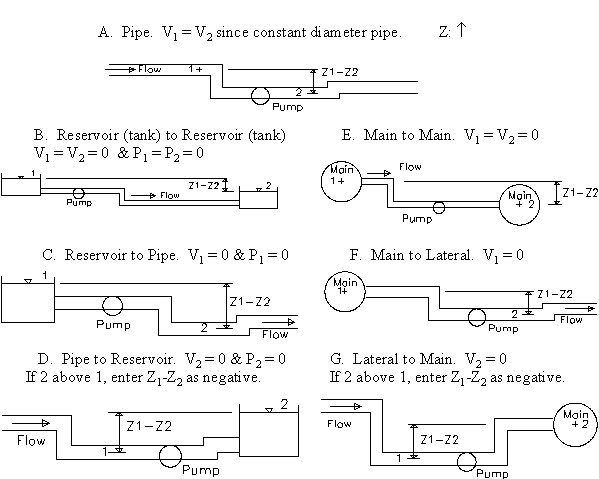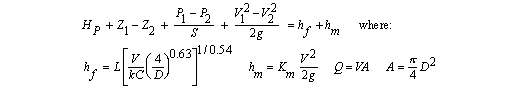Design of Circular Pressurized Water Pipes

Compute flow rate, pressure, elevation, diameter, length, minor losses, pump head in water pipes. Hazen-Williams friction loss.

Register to enable "Calculate" button.

 Flow rate, Q (cfs): Velocity: Vpipe (ft/s): Pipe Diameter, D (inch): Select Calculation & Scenario: Pipe Area, A (ft2): Solve for V, Q Q known. Solve for Z1-Z2 Q known. Solve for P1-P2 Q known. Solve for Pipe Diameter Q known. Solve for Pipe Length Q known. Solve for C Q known. Solve for Km Q known. Solve for Pump Head V known. Solve for Z1-Z2 V known. Solve for P1-P2 V known. Solve for Pipe Diameter V known. Solve for Pipe Length V known. Solve for C V known. Solve for Km V known. Solve for Pump Head Length, L (ft): A - Pipe Only B - Reservoir to Reservoir C - Reservoir to Pipe D - Pipe to Reservoir E - Main to Main F - Main to Lateral G - Lateral to Main Hazen-Williams Coefficient, C: Select Units: Minor Loss Coefficient, Km: Flow in m3/s Flow in ft3/s (cfs) Flow in U.S.gal/min (gpm) Flow in U.S.gal/day (gpd) Elevation Difference, Z1-Z2 (ft): Velocity in m/s Velocity in ft/s Pressure Diff., P1-P2 (psi): Diameter in m Diameter in cm Diameter in ft Diameter in inch Pump Head, Hp (ft): L, Z, Hp, DH, hf, hm in m. A in m2 L, Z, Hp, DH, hf, hm in ft. A in ft2 Pump Power (hp): Pressure in N/m2 (Pa) Pressure in lb/ft2 (psf) Pressure in lb/in2 (psi) Driving Head, DH (ft): Power in N-m/s (Watt) Power in ft-lb/s Power in horsepower (hp) Major (friction) Loss, hf (ft): © 2014 LMNO Engineering, Minor Loss, hm (ft): Research, and Software, Ltd. http://www.LMNOeng.com

Units in pipe design using Hazen-Williams friction loss equation: cfs=cubic foot per second, cm=centimeter, ft=foot, gal=U.S. gallon, gpd=U.S. gallon per day, gpm=U.S. gallon per mimute, hp=horsepower, lb=pound, m=meter, min=minute, N=Newton, Pa=Pascal, psi=lb/inch2, s=second

Introduction to Pipe Design using Hazen-Williams Friction Losses
This calculation is valid for water flowing at typical temperatures found in municipal water supply systems (40 to 75 oF; 4 to 25 oC). Our calculation is based on the steady state incompressible energy equation utilizing Hazen-Williams friction losses as well as minor losses. The Hazen-Williams friction loss equation is commonly used by civil engineers for municipal water distribution system design. Our pipe flow calculation can compute flowrate, velocity, pipe diameter, elevation difference, pressure difference, pipe length, minor loss coefficient, and pump head (total dynamic head).

Piping Scenarios
Since boundary conditions affect the flow characteristics, our calculation allows you to select whether your locations 1 and 2 are within pipes, at the surface of open reservoirs, or in pressurized mains (same as pressurized tank). If there is no pump between locations 1 and 2, then enter the pump head (Hp) as 0.Steady State Energy Equation used for Water Flow Through a Pipe        Back to Calculation
The first equation shown is the steady state energy equation for incompressible flow. The left side of the equation contains what we call the driving head. These heads include heads due to a pump (if present), elevation, pressure, and velocity. The terms on the right side are friction loss and minor losses. Friction losses are computed using the Hazen-Williams friction loss equation. The energy equation is well-accepted in the field of fluid mechanics and can be found in many references such as Cimbala and Cengel (2008), Munson et al. (1998), and Streeter et al. (1998), while the Hazen-Williams equation for friction losses is well-established in the water supply literature and can be found in references such as Viessman and Hammer (1998) and Mays (1999).The Hazen-Williams equation (the hf =... equation) is empirical and requires that you use particular units as noted below. Though the other equations are dimensionally correct, only units that can be used in all of the equations are shown below. Our calculation allows you to enter a variety of units and automatically performs the unit conversions.
ft=foot, lb=pound, m=meter, N=Newton, s=second

A = Pipe cross-sectional area, ft2 or m2.
C = Hazen-Williams pipe roughness coefficient. See table below for values.
D = Pipe diameter, ft or m.
Driving Head (DH) = left side of the first equation (or right side of the equation), ft. This is not total dynamic head.
g = acceleration due to gravity = 32.174 ft/s2 = 9.8066 m/s2.
hf = Major (friction) losses, ft or m.
hm = Minor losses, ft or m.
Hp = Pump head (also known as Total Dynamic Head), ft or m.
k = unit conversion factor = 1.318 for English units = 0.85 for Metric units
Km = Sum of minor loss coefficients. See table below.
Pump Power (computed by program) = SQHp, lb-ft/s or N-m/s. Theoretical pump power. Does not include an inefficiency term. Note that 1 horsepower = 550 ft-lb/s.
P1 = Upstream pressure, lb/ft2 or N/m2.
P2 = Downstream pressure, lb/ft2 or N/m2.
Q = Flow rate in pipe, ft3/s or m3/s.
S = Weight density of water  = 62.4 lb/ft3 for English units = 9800 N/m3 for Metric units
V = Velocity in pipe, ft/s or m/s.
V1 = Upstream velocity, ft/s or m/s.
V2 = Downstream velocity, ft/s or m/s.
Z1 = Upstream elevation, ft or m.
Z2 = Downstream elevation, ft or m.

All of the calculations on this page have analytic (closed form) solutions except for "Solve for V, Q" and "Q known. Solve for Pipe Diameter." These two calculations required a numerical solution. Our solution utilizes a modified implementation of Newton's method that finds roots of the equations with the result accurate to 8 significant digits. All of the calculations utilize double precision.

Table of Hazen-Williams Coefficients (C is unit-less)       Back to Calculation
Compiled from References

 Material C Material C Asbestos Cement 140 Copper 130-140 Brass 130-140 Galvanized iron 120 Brick sewer 100 Glass 140 Cast-Iron: Lead 130-140 New, unlined 130 Plastic 140-150 10 yr. old 107-113 Steel: 20 yr. old 89-100 Coal-tar enamel lined 145-150 30 yr. old 75-90 New unlined 140-150 40 yr. old 64-83 Riveted 110 Concrete/Concrete-lined: Steel forms 140 Tin 130 Wooden forms 120 Vitrif. clay (good condition) 110-140 Centrifugally spun 135 Wood stave (avg. condition) 120

Table of Minor Loss Coefficients (Km is unit-less)       Back to Calculation
Compiled from References

 Fitting Km Fitting Km Valves: Elbows: Globe, fully open 10 Regular 90o, flanged 0.3 Angle, fully open 2 Regular 90o, threaded 1.5 Gate, fully open 0.15 Long radius 90o, flanged 0.2 Gate 1/4 closed 0.26 Long radius 90o, threaded 0.7 Gate, 1/2 closed 2.1 Long radius 45o, threaded 0.2 Gate, 3/4 closed 17 Regular 45o, threaded 0.4 Swing check, forward flow 2 Swing check, backward flow infinity Tees: Line flow, flanged 0.2 180o return bends: Line flow, threaded 0.9 Flanged 0.2 Branch flow, flanged 1.0 Threaded 1.5 Branch flow, threaded 2.0 Pipe Entrance (Reservoir to Pipe): Pipe Exit (Pipe to Reservoir) Square Connection 0.5 Square Connection 1.0 Rounded Connection 0.2 Rounded Connection 1.0 Re-entrant (pipe juts into tank) 1.0 Re-entrant (pipe juts into tank) 1.0

Common Questions             Back to Calculation
I took fluid mechanics a long long time ago. What is head? Why does it have units of length? Head is energy per unit weight of fluid (i.e. Force x Length/Weight = Length).The program on this page solves the energy equation (shown below); we call energy "head."
Why is Pressure=0 for a reservoir?
A reservoir is open to the atmosphere, so its gage pressure is zero.
Why is Velocity=0 for a reservoir? This is a common assumption in fluid mechanics and is based on the fact that a reservoir has a large surface area. Therefore, the water level drops very little even if a lot of water flows out of the reservoir. A reservoir may physically be a lake or a large diameter tank.
What is a "main" and a "lateral"? A "main" is a large diameter water supply pipe that has many smaller diameter "laterals" branching off of it to supply water to individual residences, businesses, or sub-divisions. In fluid mechanics, we set V=0 for the main since it has a large diameter (relative to the lateral) and thus a very small velocity. To further justify the V=0 assumption, the main's pressure is typically high, so the velocity head in the main is negligible. The main is drawn such that it is coming out of your computer monitor.
Can I model flow between two reservoirs using either Scenario B or E? Yes, you can. If using Scenario E, just set P1-P2=0. Scenario B automatically sets P1-P2=0.
Can I model flow between two mains using either Scenario B or E? Only if the pressure is the same in both mains.
How do I model a pipe discharging freely to the atmosphere? Use Scenario A, C, or F. Since P2=0 (relative to atmospheric pressure), P1-P2 that is input or output will be P1.
What are minor losses? Minor losses are head (energy) losses due to valves, pipe bends, pipe entrances (for water flowing from a tank to a pipe), and pipe exits (water flowing from a pipe to a tank), as opposed to a major loss which is due to the friction of water flowing through a length of pipe. Minor loss coefficients (Km) are tabulated below. For our program, all of the pipes have the same diameter, so you can add up all your minor loss coefficients and enter the sum in the Minor Loss Coefficient input box.
I'm confused about pumps. Only input Pump Head if the pump is between points 1 and 2. Otherwise, enter 0 for Pump Head.
Your program is great! What are its limitations? Pipes must all have the same diameter. Pump curves cannot be implemented. The fluid must be water.
Where can I find additional information? References
What is Driving Head? See above definitions of variables. It is not total dynamic head. Hp is pump head (also known as total dynamic head).

References                 Back to Calculation
Cimbala, John M. and Yunus A. Cengel. 2008. Essentials of Fluid Mechanics: Fundamentals and Applications. McGraw-Hill.

Mays, Larry W, ed. 1999. Hydraulic Design Handbook. McGraw-Hill.

Munson, Bruce R. Donald F. Young, and Theodore H. Okiishi. 1998. Fundamentals of Fluid Mechanics. John Wiley and Sons. Inc. 3ed.

Streeter, Victor L., E. Benjamin Wylie, and Keith W. Bedford. 1998. Fluid Mechanics. McGraw-Hill. 9ed.

Viessman, Warren and Mark J. Hammer. 1998. Water Supply and Pollution Control. Addison Wesley. 6ed.

LMNO Engineering, Research, and Software, Ltd.
7860 Angel Ridge Rd.   Athens, Ohio 45701  USA   Phone: (740) 592-1890
LMNO@LMNOeng.com    http://www.LMNOeng.com

To:

Other single pipe calculators:

Hazen-Williams with pump curve

Darcy-Weisbach without pump curve

Darcy-Weisbach with pump curve

Fire hydrant test analysis

Multiple pipes:

Pipe Network

Other information:

Unit Conversions

Videos of experiment and analysis of friction loss

Register